Reach Us+44-1522-440391
A New Robust Method for Nonlinear Regression | OMICS International
Journal of Biometrics & Biostatistics

Like us on:

All submissions of the EM system will be redirected to Online Manuscript Submission System. Authors are requested to submit articles directly to Online Manuscript Submission System of respective journal.

# A New Robust Method for Nonlinear Regression

Tabatabai MA1, Kengwoung-Keumo JJ2, Eby WM3, Bae S4, Manne U5, Fouad M4 and Singh KP4*

1School of Graduate Studies and Research, Meharry Medical College, Nashville, TN 37208, USA

2Department of Mathematical Sciences, Cameron University, Lawton, OK 73505, USA

3Department of Mathematics, New Jersey City University, Jersey City, NJ 07305, USA

4Department of Medicine Division of Preventive Medicine and Comprehensive Cancer Center, University of Alabama Birmingham, Birmingham, AL 35294, USA

5Department of Pathology and Comprehensive Cancer Center, University of Alabama Birmingham, Birmingham, AL 35294, USA

*Corresponding Author:
Singh KP
Department of Medicine Division of Preventive Medicine and Comprehensive Cancer Center
University of Alabama Birmingham, Birmingham, AL 35294, USA
Tel: +205-934-6887
E-mail: [email protected]

Received date: June 30, 2014; Accepted date: September 10, 2014; Published date: September 18, 2014

Citation: Tabatabai MA, Kengwoung-Keumo JJ, Eby WM, Bae S, Manne U, et al. (2014) A New Robust Method for Nonlinear Regression. J Biom Biostat 5:199. doi: 10.4172/2155-6180.1000199

Copyright: © 2014 Tabatabai MA, et al. This is an open-access article distributed under the terms of the Creative Commons Attribution License, which permits unrestricted use, distribution, and reproduction in any medium, provided the original author and source are are credited.

Visit for more related articles at Journal of Biometrics & Biostatistics

#### Abstract

Background: When outliers are present, the least squares method of nonlinear regression performs poorly. The main purpose of this paper is to provide a robust alternative technique to the Ordinary Least Squares nonlinear regression method. This new robust nonlinear regression method can provide accurate parameter estimates when outliers and/or influential observations are present.

Method: Real and simulated data for drug concentration and tumor size-metastasis are used to assess the performance of this new estimator. Monte Carlo simulations are performed to evaluate the robustness of our new method in comparison with the Ordinary Least Squares method.

Results: In simulated data with outliers, this new estimator of regression parameters seems to outperform the Ordinary Least Squares with respect to bias, mean squared errors, and mean estimated parameters. Two algorithms have been proposed. Additionally and for the sake of computational ease and illustration, a Mathematica program has been provided in the Appendix.

Conclusion: The accuracy of our robust technique is superior to that of the Ordinary Least Squares. The robustness and simplicity of computations make this new technique more appropriate and useful tool for the analysis of nonlinear regressions.

#### Keywords

Robust nonlinear regression; Least Square estimator; Growth models; Tumor size; Metastasis; Monte-carlo simulation

#### Background

Nonlinear regression is one of the most popular and widely used models in analyzing the effect of explanatory variables on a response variable and it has many applications in biomedical research. With the presence of outliers or influential observations in the data, the ordinary least squares method can result in misleading values for the parameters of the nonlinear regression and the hypothesis testing, and predictions may no longer be reliable. The main purpose of robust nonlinear regression is to fit a model to the data that gives resilient results in the presence of influential observations, leverage points and/or outliers. Rousseeuw and Leroy  defined vertical outliers as those data points with outlying values in the direction of the response variable, while leverage points are outliers in the direction of covariates. An observation may be influential if its removal would significantly alter the parameter estimates. Edgeworth  proposed the Least Absolute Deviation as a robust method. Huber  introduced the method of M-estimation. Rousseeuw  introduced the Least Trimmed Squaresestimates. The S-estimator was introduced by Rousseeuw and Yohai . Yohai and Zammar  introduced the τ-estimator of linear regression coefficients. It is a high efficiency estimator and has a high breakdown point. Tabatabai and Argyros  extended the τ-estimates to the nonlinear regression models. Stromberg  introduced algorithms for Yohai’s MM estimator of nonlinear regression and Rousseeuw’s least median estimators of nonlinear regression. Tabatabai et al.  introduced the TELBS robust linear regression method.

In Medical, biological and pharmaceutical research and development nonlinear regression analysis has been a major tool for investigating the effect of multiple explanatory variables on a response variable when the data follows a nonlinear pattern. When outliers and influential observations are present, nonlinear least squares performs poorly. In this paper we introduce a new robust nonlinear regression method capable of handling such cases. Minn et al.  showed that lung tumor size can lead to metastasis. Also, aggressive tumor growth is a marker for cells destined to metastasize. They validated their statement by analyzing the lung metastasis gene-expression signature using a nonlinear model. Arisio et al.  study of breast cancer confirmed that the size of tumor is an important predictor of axillary lymph node metastases. Ramaswamy, et al. ) found that geneexpression signature is a significant factor associated with metastasis in solid tumors carrying such gene expressions. Maffuz et al.  showed that pure ductal carcinoma in situ is not associated with lymphatic metastasis independently of tumor size. Hense et al.  found that the occurrence and primary metastases in Ewing tumors is related to tumor size, pelvic site and malignant peripheral neuroectodermal tumors. Umbreit et al.  studied a group of patients who had undergone surgical resection for a unilateral, sporadic renal tumor. They concluded that tumor size is significantly associated with metastasis in patients suffering from renal masses. Wu et al.  retrospectively analyzed 666 patients with nasopharyngeal carcinoma and concluded that the tumor volume was correlated with cervical lymph node metastasis as well as distant metastasis after radiation therapy. In computer vision, robust regression methods have been used extensively to estimate surface model parameters in small image regions and imaging geometry of multiple cameras. Coras et al.  used nonlinear regression and showed that micromolar doses of peroxisome prolefector-activated receptor γ reduce glioma cell proliferation.

Roth  applied nonlinear sigmoidal curves to monitor the accumulation of polymerase chain reaction products at the end of each cycle by fluorescence. In human blood samples, Kropf et al.  found a nonlinear binding association between transforming growth factor beta1 (TGF -β1) and α2-Macroglobulin as well as TGF-β1 and latencyassociated peptide (LAP). Yang and Richmond  used nonlinear least squares to estimate the effective concentration of unlabeled human interferon-inducible protein 10 that yields 50% maximal binding of iodinated protein 10 to chemokine receptor CXCR3. Hao et al.  examined the significance of Nav1.5 protein in cellular processes by applying a nonlinear regression which relates the gene expression of Nav 1.5 protein and TGF-β1 as well as Nav 1.5 protein and vimentin. TGF–β families are important factors in regulation of tumor initiation, progression, and metastatic activities, Bierie, et al. . Coras et al.  applied nonlinear regression models to show that traglitazone concentration has a tendency to inhibit 1 TGF-β1 release in glioma cell culture.

This paper introduces a new robust nonlinear regression estimator. This new method for robust nonlinear regression has a bounded influence and high breakdown point and asymptotic efficiency under normal distribution and is able to estimate the parameters of nonlinear regression in such a way that is close to the parameter estimates we would have estimated with the absence of outliers in the data. In addition, this new robust nonlinear regression method is computationally simple enough to be used by practitioners.

#### Materials and Methods

We begin with the introduction of our new robust nonlinear regression model. The introduction of the model is followed by two algorithms describing its implementation. We then apply this new model to a real data set with an outlier present. In addition, we will analyze a problem involving tumor size and metastases with and without outliers. Monte Carlo simulations are also performed to evaluate the robustness of our method, in comparison with the ordinary least squares method.

Robust nonlinear regression model

Consider the general nonlinear model of the form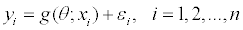Where y1, y2,..., yn is a sample of n observations with k predictor variables in the model and the parameter vector θ=(θ1, θ2,..., θp). The errors εi’s are random variables. In a designed experiment, xij’s are fixed but when xij’s are observational, they are random variables. The predictor can be fixed, random, or mixed. The ordinary least squares estimate of the parameter vector θ is given by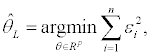and the robust estimate of the parameter vector θ is derived by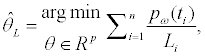(1)

where the function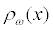is defined as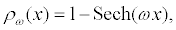and the positive real number ω is called the tuning constant. The function Sech (•) is the hyperbolic secant function and ti ’s are defined by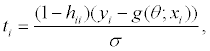(2)

where σ is the error standard deviation and hii’s are the diagonal elements of the matrix H of the form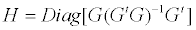where the matrix G is defined as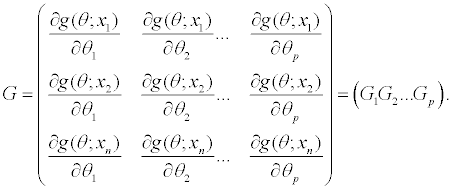For j =1,2,...,k, we define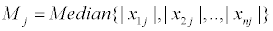and for i =1,2,..., n, we define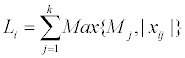If σ is unknown, one may use one of the following two estimators of σ which were proposed by Rousseeuw and Croux .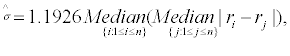Or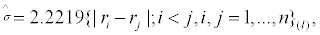(4)

where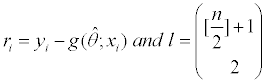is the binomial coefficient and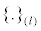is the l th order statistic.

The above estimators of σ have high breakdown points. Under the normality assumption for error terms, the estimators given in (3) and (4) have higher efficiency than median absolute deviation (MAD). In this paper all of our computations are performed using formula (3).

The function ρω: R→R is a differentiable function satisfying the following properties:

i. ρω(0)=0,

ii. ρω is bounded,

iii. ∀x∈R, ρω(x) ≥ 0,

iv. ∀x∈R, ρω(x)=ρω (-x),

v.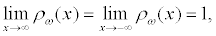vi.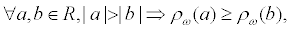vii.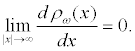viii.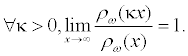Taking the partial derivatives of (1) with respect to parameters and setting them equal to zero results in the following system of equations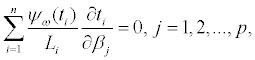(5)

where ψω is the derivative of ρω which is equal to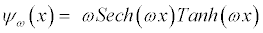Define the weights wi as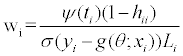(6)

Then for j =1, 2,..., p, the equation (5) can be written as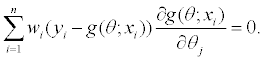The matrix of weights, W is a diagonal matrix whose elements on the main diagonals are w1,w2,...,wn, and the estimator of the parameter vector θ is given by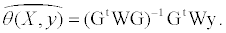If g(θ;xi) is linear function of parameters, then the above model would be identical to TELBS robust linear regression model. Asymptotically,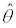has a normal distribution with mean θ and variancecovariance matrix of the form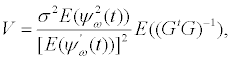where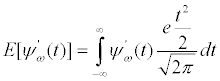and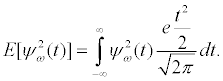The function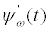is defined as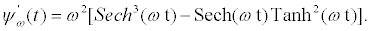Under the assumption of normality for the underlying distribution, the asymptotic efficiency, Aeff, is defined as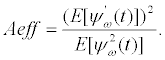The tuning constant ω can be calculated by solving equation (7) for ω.

An estimate for the variance-covariance matrix is derived and given as followsThe robust deviance is defined as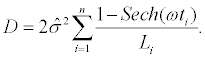The deviance plays a major role in model fitting. A smaller value of deviance is preferred over larger values. Following Akaike Criterion  and Ronchetti , the robust equivalence of AIC is denoted by AICR, and is given by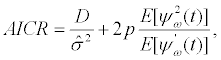and the Robust Schwarz Information Criterion BICR is given by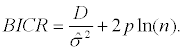A robust coefficient of determination is given by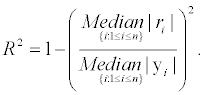For more details, see Rosseeuw and Leroy .

There are numerous variable selection techniques available in the literature. One may use the stepwise procedure that may involve in forward selection or backward elimination. For each set S ⊆{x1, x2..., xp} of explanatory variables, the robust final predicted error of Maronna et al.  is denoted by RFPE(S) and is defined as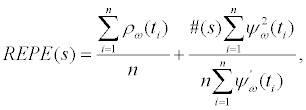where #(S) is the number of elements in the set S. In the forward selection or backward elimination, choose the one whose inclusion or deletion results in the smallest value of RFPE. To perform hypothesis testing, we let Ω⊆ R be the parameter space and {θj1j2,...,θjq} be asubset of {θ12,...,θp}.

Define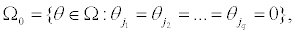and the function f (θ) as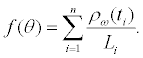Then a robust likelihood ratio type test statistic for testing the null hypothesis H0:θ∈Ω0 against the alternative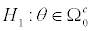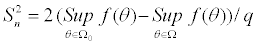For more information, the reader is referred to Hampel et al. . Asymptotically under the null hypothesis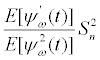has a chisquare distribution with q degrees of freedom, where the Wald type test statistic is defined as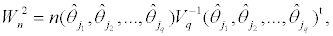and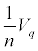is the asymptotic variance-covariance matrix for the given vector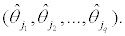the null distribution of the statistic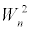is asymptotically a chi-square distribution with q degrees of freedom.

Any of the following two robust algorithms can be used to estimate the parameter vector θ and standard deviation σ of a nonlinear regression model:

Algorithm I:

1. Set j=0 and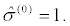Calculate the initial estimate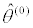of parameter vector θ by minimizing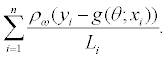2. Set j=j+1 and calculate the followings :

i.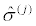using formula (3) or (4).

ii. Use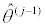to evaluate matrix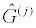.

iii. Evaluate matrix H(j) =Diagonal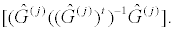iv. The ith diagonal element of matrix H(j) is denoted by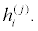Calculate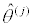by minimizing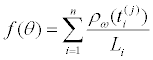, where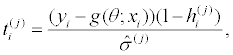If convergence occurs, stop. Otherwise go to step 2 and continue the process.

The convergence occurs when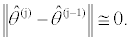The convergence criterion is to stop the algorithm when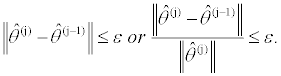One may choose the value of ε=0.00001.

Algorithm II:

1. Set j=0 andCalculate the initial estimateof parameter vector θ by minimizing2. Set j=j+1. For 1≤ i ≤ n, calculate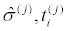and weights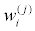where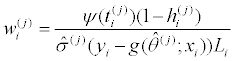anduse them to calculate the weights matrix w(j).

3. Use information from step 2 to calculate.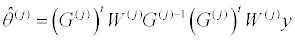4. If convergence occurs, stop. Otherwise go to step 2 and continue the process.

The convergence occurs whenThe convergence criterion is to stop the algorithm whenOne may choose the value of ε=0.00001.

Drug concentration data

Kenakin  used a set of responses to the concentration of an agonist in a functional assay. They fit the following model to their data. In this data, observation 5 has an outlier in the response direction,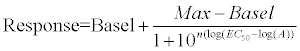(8)

Table 1 shows their actual and predicted concentrations as well as our results for fitted Hyperbolastic model of type III (H3). For this example, the new robust technique is an effective regression tool in estimating model parameters in the presence of outliers. Figure 1 shows the fitted curve using hyperbolastic model of type III (H3). Figure 2 uses formula (8) and the least squares fitted curve for the concentration data.

OBS Concentration Response Least Square Calculating using 1 Hyperbolastic H3
1 0.01 2 2 0.8 2
2 0.03 8 8.7 5.2 8
3 0.1 28 27.8 28 27.9
4 0.3 59 59.0 62.6 61.4
5 1 95 84.3 77.9 78.1
6 3 78 84.3 80.4 78.4
7 10 80 84.3 80.8 78.4

Table 1: Parameter Estimates for the Concentration Data.

Tumor metastasis

The data in Table 2 consist of 12 observations. The response variable is the fraction of breast cancer patients with metastases and the predictor variable is the tumor size. Table 2 is from Michaleson et al. . This data was originally collected by Tabar et al. [30-32] and Tubiana et al. [33,34]. To assess the robustness of our new method with regard to a special class of nonlinear growth models, we utilize this tumor metastasis data that is free of outliers. We first fit a model to the data using the robust method as well as least squares when there is no outlier present. Then we plant outliers in X direction, Y direction and both X and Y direction. In the X direction we change the X value in observation 12 from 90 to 2. In the Y direction, we change the y value in observation 6 from .55 to 3 and in both X and Y direction we change observation 12 in X direction from 90 to 2 and observation 7 in Y from .56 to 3.

 Tumor size x 12 17 17 25 30 39 40 50 60 70 80 90 Fraction Metastasized y 0.13 0.2 0.27 0.45 0.42 0.55 0.56 0.66 0.78 0.83 0.81 0.92

Table 2: Tumor Size Versus Fraction Metastasized Data.

For illustrative purposes, we have fitted hyperbolastic of type II, Gompertz and logistic models. In the past, these models have been used to monitor cancer progression and regression. Each model has three parameters θ1, θ2, and θ3 with θ1 and θ2 being positive and εi are random errors. The response yi is the fraction metastasized and xi is the tumor size for individual i. The left graphs in Figures 3-5 are fitted curves using our proposed robust nonlinear regression technique and the graphs on the right sides of Figures 3-5 have been drawn using the nonlinear least square regression technique by planting outliers in X direction, Y direction and both X and Y direction. As you can see, when there is no outlier in the data all models perform well regardless of using the robust method or Least Squares. But when we plant outliers in the X, Y, and/or XY directions the fits become unacceptable for Least Squares whereas the robust method performs well for all models.

The hyperbolastic model of type II or simply H2 has the form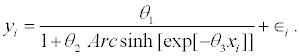The Gompertz model is of the form: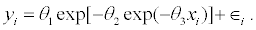The logistic model is of the form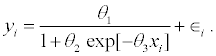Simulation

We perform simulation experiments to evaluate the robustness of our new nonlinear regression method compared to the least squares method. We have simulated the biochemistry model known as Michaelis-Menten kinetics. In biochemistry this model expresses the reaction velocity V as a function of concentration of substrate C as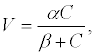where the parameter α denotes the maximum reaction velocity and β is the substrate concentration at which the initial velocity V0 is 50% of the maximum reaction velocity. The larger the parameter β, the lower is the efficiency between the substrate and enzyme. This model has also been used in many biological systems such as gene regulatory system. In order to investigate the robustness of our new method relative to the method of least squares, we considered the nonlinear Michaelis- Menten equation of the form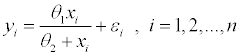Where the response variable is yi and xi is fixed. In our simulations we set xi=i and εi as the standard normal distribution with mean 0 and standard deviation 1. We performed 1000 repetitions using two sample sizes n=20 and n=50. The outliers were randomly chosen in the direction of X, Y and both X and Y. We used contamination levels of 0%, 10%, 20%, 30%, and 40%. In this simulation the parameter values are θ1=5 and θ2 =1. The software Mathematica is used in the simulation process. To evaluate the robustness of these estimators, we randomly choose 10%, 20%, 30% and 40% of the simulated observations and contaminate the selected data by magnifying their size by a factor of 100 in the direction of explanatory variable X, response variable Y, and both response Y and explanatory X variables. Finally, we estimate both bias and mean squared errors using the following equations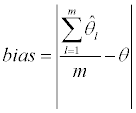Where m is the number of iterations in the simulation. The mean squared error is estimated by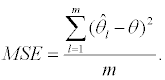Tables 3-5 give the summary of our simulation outcome for both small and large sample sizes. The asymptotic efficiency for our simulation studies has been set to 95% level. By examining the simulation tables, we find out that in the absence of contamination in the simulated data, both the least square and the proposed robust method perform well with respect to bias, mean square error and mean estimated parameter values. However, when contamination enters into our simulated data in the direction of explanatory variable X, or response variable Y, or both X and Y, then the new method outperforms the least squares method for both small and large samples. We also observe that the estimated values of parameters θ1 and θ2 are in close proximity of the true values of the parameters θ1 and θ2. The simulation results clearly indicate the robustness of our new nonlinear regression technique relative to least squares method when outliers or influential observations are present.

n=20   0% 10% 20% 30% 40%
θ1 θ2 θ1 θ2 θ1 θ2 θ1 θ2 θ1 θ2
Least-Squares Bias 0.0375 0.1083 25343 47179 8583 6953 2407 5185 1604 4015
MSE 0.0479 0.4842 5.5E+9 1.5E+10 4.6E+9 2.1E+9 3.2E+8 2.2E+9 1.0E+8 1.4E+9
MEP 5.0375 1.1083 25348 45180 9324 7329 3456 7733 1934 5234
Robust Method Bias 0.0901 0.1525 0.0056 0.2242 0.034 0.2421 0.0199 0.2265 0.0176 0.3269
MSE 0.0534 0.5646 0.2111 0.9423 0.2502 1.2292 0.2578 0.8812 0.2767 1.6324
MEP 5.0701 1.1327 5.0056 1.2201 5.0134 1.2440 5.0199 1.2263 4.9824 1.3269
n=50   0% 10% 20% 30% 40%
θ1 θ2 θ1 θ2 θ1 θ2 θ1 θ2 θ1 θ2
Least-Squares Bias 0.0131 0.1430 5214 4108 7326 5423 2113 4927 1476 3104
MSE 0.0396 0.2566 6.1E+9 1.7E+9 3.2E+9 1.8E+9 2.5E+8 1.8E+9 7.9E+7 1.2E+9
MEP 5.0131 1.0414 30123 58972 8589 6954 2412 6186 1609 4016
Robust Method Bias 0.0701 0.1625 0.0328 0.0914 0.02925 0.1285 0.0494 0.1843 0.0050 0.2876
MSE 0.2167 0.5221 0.2333 0.6257 0.2718 0.7224 0.3150 0.8543 0.2937 0.9745
MEP 5.0701 1.1131 5.0328 1.0914 5.0292 1.1285 5.0494 1.1843 4.9734 1.1567

Table 3: Bias, Mean Square Errors (MSE) and Mean Estimated Parameter (MEP) with Percentage Contamination in the X Direction.

n=20   0% 10% 20% 30% 40%
θ1 θ2 θ1 θ2 θ1 θ2 θ1 θ2 θ1 θ2
Least-Squares Bias 0.0375 0.1083 68395 12511 115870 12323 115212 9858 136703 8915
MSE 0.0479 0.4842 1.50E+10 1.50E+8 1.2E+11 1.2E+9 1.4E+11 9.9E+8 2.4E+11 1.0E+9
MEP 5.0375 1.1083 68400 12512 115875 12324 115217 9859 136708 8916
Robust Method Bias 0.0901 0.1525 0.0358 0.0523 0.0362 0.0250 0.0899 0.1605 0.2034 0.3292
MSE 0.0534 0.5646 0.2296 0.5710 0.2555 0.6212 0.3534 0.8233 0.3928 1.095
MEP 5.0701 1.1327 4.9641 1.0523 4.9638 0.9751 5.0899 1.1601 5.2034 1.3292
n=50   0% 10% 20% 30% 40%
θ1 θ2 θ1 θ2 θ1 θ2 θ1 θ2 θ1 θ2
Least-Squares Bias 0.0131 0.1430 23650 11692 18843 5605 25855 5462 33790 5170
MSE 0.0396 0.2566 4.6E+9 1.1E+9 7.6E+9 6.3E+9 1.2E+10 5.1E+08 3.1E+10 7.1E+08
MEP 5.0131 1.0414 23655 11693 18848 5606 25860 5463 33795 5171
Robust Method Bias 0.0701 0.1625 0.0365 0.0646 0.0262 0.0143 0.0504 0.0640 0.0022 0.0510
MSE 0.2167 0.5221 0.0555 0.2067 0.0660 0.3303 0.0673 0.3861 0.0710 0.6139
MEP 5.0701 1.1131 5.0365 1.0646 4.974 0.9857 5.0505 1.0650 5.0022 1.0510

Table 4: Bias, Mean Square Errors (MSE) and Mean Estimated Parameter (MEP) with Percentage Contamination in the Y Direction.

n=20   0% 10% 20% 30% 40%
θ1 θ2 θ1 θ2 θ1 θ2 θ1 θ2 θ1 θ2
Least-Squares Bias 0.0375 0.1083 25342.0 45178 8582 6952 2406 5184 1603 4015
MSE 0.0479 0.4842 5.5E+9 1.5E+10 4.6E+9 2.1E+9 3.2E+8 2.2E+9 1.0E+8 1.4E+9
MEP 5.0375 1.1083 25347 45179 8587 6953 2411 5185 1608 4016
Robust Method Bias 0.0901 0.1525 0.0762 0.0895 0.0081 0.0599 0.1191 0.1685 0.0176 0.0417
MSE 0.0534 0.5646 0.1676 0.3112 0.1484 0.6847 0.2901 0.7813 0.2539 0.7448
MEP 5.0701 1.1327 5.0762 1.0895 4.9919 1.0599 5.1191 1.1685 5.0176 0.0417
n=50   0% 10% 20% 30% 40%
θ1 θ2 θ1 θ2 θ1 θ2 θ1 θ2 θ1 θ2
Least-Squares Bias 0.0131 0.1430 660 892 583 582 566 478 559 404
MSE 0.0396 0.2566 455959 1.0E+6 345138 437874 323227 252951 314270 176481
MEP 5.0131 1.0414 665 893 588 583 571 479 564 405
Robust Method Bias 0.0701 0.1625 0.0173 0.0736 0.0892 0.1767 0.0399 0.0059 0.01445 0.0256
MSE 0.2167 0.5221 0.0526 0.3141 0.0746 0.4530 0.0474 0.2435 0.0857 0.4877
MEP 5.0701 1.1131 4.9827 1.0736 5.0892 1.1767 4.9601 1.0060 4.9856 1.0256

Table 5: Bias, Mean Square Errors (MSE) and Mean Estimated Parameter (MEP) with Percentage Contamination in the X-Y-Direction.

#### Conclusion

In this paper we introduced a new robust estimator of nonlinear regression parameters. In addition, robust testing for hypothesis about model parameters was introduced. Moreover, two algorithms were developed to perform the robust nonlinear estimation of model parameters. The computer simulation revealed the robustness of our new estimator. This robust method provides a powerful alternative to least squares method. The robust method presented in this paper has influence functions bounded in both the response and the explanatory variable direction. It has high asymptotic breakdown point and efficiency. A Mathematica program is also provided to ease in computations. This program does the necessary calculations to perform the robust nonlinear regression analysis of the drug concentration example given in this paper.

#### Acknowledgement

Research reported in this paper was partially supported by the Center grant of the National Cancer Institute of the National Institutes of Health to the University of Alabama at Birmingham Comprehensive Cancer Center (P30 CA013148), the Cervical SPORE grant (P50CA098252), the Morehouse/Tuskegee University/ UAB Comprehensive Cancer Center Partnership grant (2U54-CA118948), and the Mid-South Transdisciplinary Collaborative Center for Health Disparities Research (U54MD008176). The content is solely the responsibility of the authors and does not necessarily represent the official views of the National Institutes of Health.

#### References

Select your language of interest to view the total content in your interested language

### Article Usage

• Total views: 12713
• [From(publication date):
June-2014 - Nov 13, 2019]
• Breakdown by view type
• HTML page views : 8872Can't read the image? click here to refresh